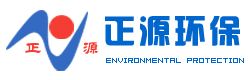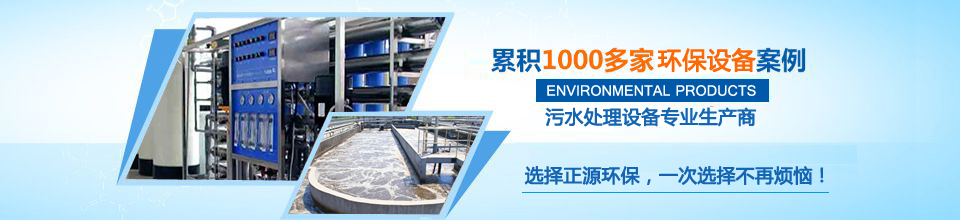#一体化净水设备、粉末活性炭投加装置全套解决方案一体化净水设备专业生产商。

13573692838
-SWB系列地埋式一体化污水处理设备

·宾馆、疗养院、医院、学校、住宅小区、别墅小区、高速公路服务区、火车站等生活污水的处理。
·多种工业有机废水。
·水产加工厂、畜牧加工厂、鲜奶加工厂等生产废水的处理。
·机械加工厂、食品加工厂、石化加工厂等生产废水的处理。

·本设备安装要求地面平整，并且地基每平方达到承重8吨以上。
·安装完毕后须把电控柜控制线与设备接通，电控柜与电源接通，接线时注意风机、水泵的转向。

·设备如需检修一般不抽空内部污水，需留有一定高度水位，以防地下水把设备浮起。
·设备初次进水注意各池液位差不要超过一米，以防设备变形。
·为保证运行出水效果，风机停止状态严禁进水。
·污泥启动，首次接种将污泥投加到水解酸化池和两个接触氧化池中，为了避免菌种流失，可减少进水量并启动风机进行曝气，直至填料上长出一层橙黄色生物膜，即完成填料挂膜，然后将进水量增加至额定流量。
·污泥回流，本设备要注意经常把二沉池污泥回流到水解酸化池中。
·系统排泥，当设备运行一段时间后，系统总污泥量较高、出水水质下降要采取排泥措施，可用真空吸粪车将污泥浓缩池污泥吸出。
·定期检查风机与水泵各部螺丝松动情况，填料的松紧情况、轴承的温度和润滑油的油质和油量，保证各部正常运行。
·风机的运行及保养。风机启动前必须注意空气阀门是否已打开。风机每运行10000小时必须保养一次。
·水泵的运行及保养。水泵要注意检查密封情况，如有漏水及时处理。与泵体相连的联接螺丝和机座螺丝做好定期坚固工作，并做防锈处理。水泵每运行5000～8000小时必须保养一次。
·风机及水泵保养祥见风机、水泵说明书。

 型号 处理量（m³/h） 风机 水泵 备注 SWB-0.5 0.5 Q=0.13m³/min，P=2.5m，N=0.75KW Q=0.5m³/h，H=8m，N=0.75 SWB -1 1 Q=0.25m³/min，P=2.5m，N=0.75KW Q=1m³/h，H=8m，N=0.75 SWB -2 2 Q=0.50m³/min，P=2.5m，N=0.75KW Q=2m³/h，H=8m，N=0.75 SWB -3 3 Q=0.75m³/min，P=2.5m，N=0.75KW Q=3m³/h，H=8m，N=0.75 SWB -4 4 Q=1.00m³/min，P=2.5m，N=0.75KW Q=4m³/h，H=8m，N=0.75 SWB -5 5 Q=1.25m³/min，P=2.5m，N=1.1KW Q=5m³/h，H=8m，N=0.75 SWB -6 6 Q=1.50m³/min，P=2.5m，N=1.5KW Q=6m³/h，H=8m，N=0.75 SWB -8 8 Q=2.00m³/min，P=2.5m，N=2.2KW Q=8m³/h，H=8m，N=0.75 SWB -10 10 Q=2.50m³/min，P=2.5m，N=2.2KW Q=10m³/h，H=8m，N=0.75 SWB -15 15 Q=3.75m³/min，P=2.5m，N=3KW Q=15m³/h，H=8m，N=0.75 SWB -20 20 Q=5.00m³/min，P=2.5m，N=4KW Q=20m³/h，H=8m，N=1.5 SWB -25 25 Q=6.25m³/min，P=3.0m，N=5.5KW Q=25m³/h，H=8m，N=2.2 SWB -30 30 Q=7.50m³/min，P=3.0m，N=7.5KW Q=30m³/h，H=8m，N=2.2 SWB -40 40 Q=10.00m³/min，P=3.0m，N=11KW Q=40m³/h，H=8m，N=3 SWB -50 50 Q=12.50m³/min，P=3.0m，N=15KW Q=50m³/h，H=8m，N=3

#### 联系山水